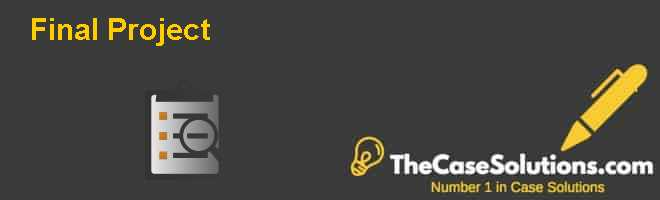# Final Project Harvard Case Solution & AnalysisConsidering that Exclusive Billiards has a combined state and federal tax rate of 30 percent. How many tables does it need to produce and sell each month to earn per year after-tax profit of \$ 60,000?

In order to earn a profit of \$60,000 after tax; the company needs to make \$5000 profit after tax per month, which is \$7,143 before tax. The company is generating 10,000 contribution per unit and sells 5 units to breakeven so it needs to make additional contribution of \$7143 to achieve the desired profit for which additional 0.71 units are needed to be sold, which leads to a total of 5.71 units to be sold to earn a profit of \$60,000 after tax.

 QUESTION-D Per unit Price per Pool 13,200 Variable Cost Per Pool 3,200 Contribution per Unit 10,000 Total Fixed Cost 50,000 Break Even Units 5 Monthly Target Profit After Tax 5,000 Tax Rate 30% Monthly Target Profit Before Tax 7,143 Additional Units Required to be sold per month in order to generate Addition contribution 0.71 Total Target Units Required to be sold 5.71 (D) Revenue 75,429 Less: Variable cost (18,286) Contribution 57,143 Less: Fixed Cost (50,000) Profit Before Tax 7,143 Tax @ 30% (2,143) Monthly Profit After Tax 5,000 Annual Profit After Tax 60,000

Estimate the operating leverage and the markup percentage considering the production for 7 tables.

Operating gearing shows the effect of fixed cost on the sales and operating profits of the company, which is high fixed cost that will lead to higher operational gearing. Therefore, it can be said that the greater the fixed cost; greater will be the operational gearing and the lower will be the operational profit.

The operating gearing of the company stands at 3.5, which means that the contribution of the company in making 7 units is 3.5 times the fixed cost of the company.

The markup charged by the company is calculated by taking the difference of total cost per unit and the selling price of the company, which currently stands at 28%. At this point, the variable cost per unit is already provided and the fixed cost per unit is calculated by 50000 total fixed costs divided by 7 units.

 QUESTION-E OPERATING GEARING CONSIDERING THE PRODUCTION OF 7 POOL TABLES OPERATING GEARING 3.5 MARKUP PERCENTAGE PRICE PER UNIT \$                             13,200.00 VARIABLE COST PER UNIT \$                               3,200.00 FIXED COST PER UNIT \$                               7,142.86 TOTAL COST PER UNIT \$                             10,342.86 MARKUP ON COST \$                               2,857.14 MARKUP PERCENTAGE ON COST 28%

Exclusive billiards is planning to extend the manufacturing unit by installing controlled automated machines in order to industrialize the production of pool tables. This will allow the production of 50 tables per month at the selling price of \$ 10,000 each. The investment cost of the machines is of \$ 8,000,000. The thirty percent of this cost can be depreciated over the useful life of the investment in accordance with a linear schedule. Estimate the Net present value the investment considering a nominal interest rate of 8%. Which is the discount payback period of the investment? Then estimate the return on investment – ROI; and the internal rate of return. Finally please discuss the economic benefits of this investment projects.

If the investment of \$8,000,000 is made by the company, which will result in increased production, then according to the discounted cash flow calculation; the NPV of the project stands at \$16.2 million. Moreover, the project is assumed to run for 20 years as that will be the life of the machinery and depreciation of the machinery, which is calculated on a straight line bases as per 20 years of life of the machinery.

If 50 tables are made per month then a total of 600 tables will be made and sold in a year, which will lead to revenue of \$6 million as per the selling price of \$10,000. In addition, the variable cost of per table is assumed to be the same as provided above that is \$3600, which will lead to a total variable cost of \$1.92 million and fixed cost is also assumed to be the same \$50,000 per month leading to \$600,000 per year whereas tax is charged at 30%.

The investment of \$8 million will be paid back in 3.9 years whereas the IRR stands at 21%, which is clearly higher than the cost of capital of the company that makes it a very viable investment and return on investment stands at 29%, which is a very good return considering the high amount of investment....................................

This is just a sample partial case solution. Please place the order on the website to order your own originally done case solution.Other Similar Case Solutions like